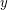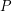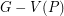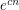# Lovasz, Laszlo

## Erdős–Faber–Lovász conjecture ★★★

Author(s): Erdos; Faber; Lovasz

Conjecture   Ifis a simple graph which is the union ofpairwise edge-disjoint complete graphs, each of which hasvertices, then the chromatic number ofis.

Keywords: chromatic number

## Lovász Path Removal Conjecture ★★

Author(s): Lovasz

Conjecture   There is an integer-valued functionsuch that ifis any-connected graph andandare any two vertices of, then there exists an induced pathwith endsandsuch thatis-connected.

Keywords:

## Double-critical graph conjecture ★★

Author(s): Erdos; Lovasz

A connected simple graphis called double-critical, if removing any pair of adjacent vertexes lowers the chromatic number by two.

Conjectureis the only-chromatic double-critical graph

Keywords: coloring; complete graph

## Exponentially many perfect matchings in cubic graphs ★★★

Author(s): Lovasz; Plummer

Conjecture   There exists a fixed constantso that every-vertex cubic graph without a cut-edge has at leastperfect matchings.

Keywords: cubic; perfect matching

## Hamiltonian paths and cycles in vertex transitive graphs ★★★

Author(s): Lovasz

Problem   Does every connected vertex-transitive graph have a Hamiltonian path?

Keywords: cycle; hamiltonian; path; vertex-transitive